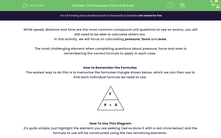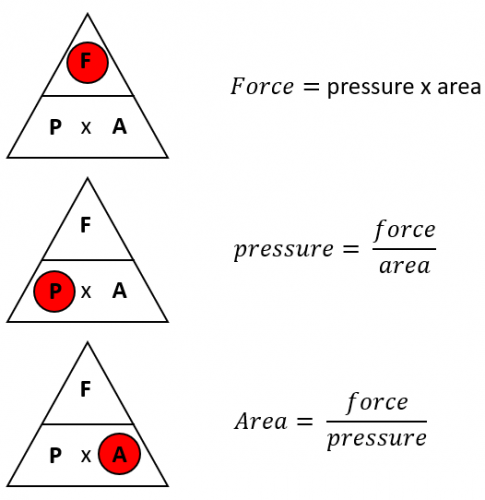# Find Pressure, Force and Area

In this worksheet, students will practise using the Force, Pressure, Area formulae pyramid to find the correct single formula to apply to find a missing variable.Key stage:  KS 4

Year:  GCSE

GCSE Subjects:   Maths

GCSE Boards:   AQA, Eduqas, Pearson Edexcel, OCR,

Popular topics:   Area worksheets

Difficulty level:#### Worksheet Overview

While speed, distance and time are the most common compound unit questions to see on exams, you will still need to be able to calculate others too.

In this activity, we will focus on calculating pressure, force and area.

The most challenging element when completing questions about pressure, force and area is remembering the correct formula to apply in each case.

How to Remember the Formulae

The easiest way to do this is to memorise the formulae triangle shown below, which we can then use to find each individual formula we need to use:How To Use This Diagram

It's quite simple, just highlight the element you are seeking (we've done it with a red circle below) and the formula to use will be constructed using the two remaining elements.Let's look at this formulae in action now in some examples.

e.g What is the pressure when a force of 8 N is applied to an area of 2 m2?

The first step in these calculations is always to decide what we need to find out.

In this case, we are directly asked for the to find pressure, so we need to use this formula:

P = F ÷ A

Now we can simply plug our numbers from the question into this formula:

P = 8 ÷ 2

The last question that we need to ask ourselves is which units to use with our answer.

Pressure here could be given in N/m2, but could also be given in Pascals (Pa).

These are both viable options and you will get marks either way.

So Pressure = 4 Pa or 4 N/m2

e.g. The pressure on an object is 3 Pa and the force being applied is 15 N. What is the area on which the force is acting?

Once again, we need to decide which formula to use:

A = F ÷ P

Plugging in our numbers gives us:

A = 15 ÷ 3 = 5

When you are dealing with pressure, force and area, unless you are told otherwise, the units to use will be m2.

So Area = 5 m2

In this activity, we will use the Force, Pressure, Area formulae pyramid to find the correct single formula to apply to find a missing variable using the method shown above.

### What is EdPlace?

We're your National Curriculum aligned online education content provider helping each child succeed in English, maths and science from year 1 to GCSE. With an EdPlace account you’ll be able to track and measure progress, helping each child achieve their best. We build confidence and attainment by personalising each child’s learning at a level that suits them.

Get started